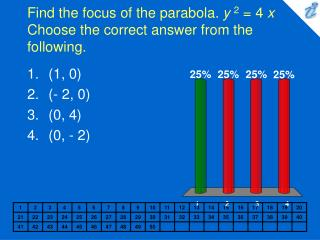Download PresentationFind the focus of the parabola. y 2 = 4 x Choose the correct answer from the following.

# Find the focus of the parabola. y 2 = 4 x Choose the correct answer from the following. - PowerPoint PPT PresentationDownload Presentation## Find the focus of the parabola. y 2 = 4 x Choose the correct answer from the following.

- - - - - - - - - - - - - - - - - - - - - - - - - - - E N D - - - - - - - - - - - - - - - - - - - - - - - - - - -
##### Presentation Transcript

1. Find the focus of the parabola. y2 = 4 x Choose the correct answer from the following. • (1, 0) • (- 2, 0) • (0, 4) • (0, - 2)

2. Find an equation for the parabola, that has a focal diameter 36 and the focus on the negative y-axes. Choose the correct answer from the following. • x2 = - 36y • x2 = 36y • x2 = - 9y • y2 = 9x

3. Find the vertices of the ellipse. {image} . Choose the correct answer from the following: • (-10, 0) and (10, 0) • (-6, 0) and (6, 0) • (-8, 0) and (8, 0) • None of these choices

4. The ancillary circle of an ellipse is the circle with radius equal to half the length of the minor axis and a center the same as the ellipse. The ancillary circle is thus the largest circle that can fit within an ellipse. Find an equation for the ancillary circle of the ellipse x2 + 4 y2 = 16. Choose the correct answer from the following. • x2 + y2 = 4 • x2 + y2 = 16 • x2 + y2 = 1 • x2 + y2 = 9 • None of these choices

5. 1. 2. 3. 4. Find the equation for the hyperbola with foci {image} and vertices {image} . Choose the answer from the following: • {image} • {image} • {image} • {image}

6. Find an equation for the conic whose graph is shown. {applet} Choose the answer from the following: • y2 = 5.6(x + 4) • y2 = 16(x + 1.4) 2 • x2 = 5.6(y - 4) • y = 5.6(x + 4) 2

7. 1. 2. 3. Determine the equation of the conic {image} in XY-coordinates when the coordinate axes are rotated through the angle {image} Choose the answer from the following : • {image} • {image} • {image}

8. 1. 2. 3. Use a rotation of axes to eliminate the xy-term in the equation {image} Choose the answer from the following : • {image} • {image} • {image}

9. 1. 2. 3. 4. Write a polar equation of an ellipse that has eccentricity 0.7 and vertex at the point {image} Choose the answer from the following : • {image} • {image} • {image} • {image}